Calculus Volume 1

# 4.6Limits at Infinity and Asymptotes

Calculus Volume 14.6 Limits at Infinity and Asymptotes

### Learning Objectives

• 4.6.1 Calculate the limit of a function as $x x$ increases or decreases without bound.
• 4.6.2 Recognize a horizontal asymptote on the graph of a function.
• 4.6.3 Estimate the end behavior of a function as $x x$ increases or decreases without bound.
• 4.6.4 Recognize an oblique asymptote on the graph of a function.
• 4.6.5 Analyze a function and its derivatives to draw its graph.

We have shown how to use the first and second derivatives of a function to describe the shape of a graph. To graph a function $ff$ defined on an unbounded domain, we also need to know the behavior of $ff$ as $x→±∞.x→±∞.$ In this section, we define limits at infinity and show how these limits affect the graph of a function. At the end of this section, we outline a strategy for graphing an arbitrary function $f.f.$

### Limits at Infinity

We begin by examining what it means for a function to have a finite limit at infinity. Then we study the idea of a function with an infinite limit at infinity. Back in Introduction to Functions and Graphs, we looked at vertical asymptotes; in this section we deal with horizontal and oblique asymptotes.

#### Limits at Infinity and Horizontal Asymptotes

Recall that $limx→af(x)=Llimx→af(x)=L$ means $f(x)f(x)$ becomes arbitrarily close to $LL$ as long as $xx$ is sufficiently close to $a.a.$ We can extend this idea to limits at infinity. For example, consider the function $f(x)=2+1x.f(x)=2+1x.$ As can be seen graphically in Figure 4.40 and numerically in Table 4.2, as the values of $xx$ get larger, the values of $f(x)f(x)$ approach $2.2.$ We say the limit as $xx$ approaches $∞∞$ of $f(x)f(x)$ is $22$ and write $limx→∞f(x)=2.limx→∞f(x)=2.$ Similarly, for $x<0,x<0,$ as the values $|x||x|$ get larger, the values of $f(x)f(x)$ approaches $2.2.$ We say the limit as $xx$ approaches $−∞−∞$ of $f(x)f(x)$ is $22$ and write $limx→-∞f(x)=2.limx→-∞f(x)=2.$

Figure 4.40 The function approaches the asymptote $y=2y=2$ as $xx$ approaches $±∞.±∞.$
 $xx$ $1010$ $100100$ $1,0001,000$ $10,00010,000$ $2+1x2+1x$ $2.12.1$ $2.012.01$ $2.0012.001$ $2.00012.0001$ $xx$ $−10−10$ $−100−100$ $−1000−1000$ $−10,000−10,000$ $2+1x2+1x$ $1.91.9$ $1.991.99$ $1.9991.999$ $1.99991.9999$
Table 4.2 Values of a function $f f$ as $x → ± ∞ x → ± ∞$

More generally, for any function $f,f,$ we say the limit as $x→∞x→∞$ of $f(x)f(x)$ is $LL$ if $f(x)f(x)$ becomes arbitrarily close to $LL$ as long as $xx$ is sufficiently large. In that case, we write $limx→∞f(x)=L.limx→∞f(x)=L.$ Similarly, we say the limit as $x→−∞x→−∞$ of $f(x)f(x)$ is $LL$ if $f(x)f(x)$ becomes arbitrarily close to $LL$ as long as $x<0x<0$ and $|x||x|$ is sufficiently large. In that case, we write $limx→−∞f(x)=L.limx→−∞f(x)=L.$ We now look at the definition of a function having a limit at infinity.

### Definition

(Informal) If the values of $f(x)f(x)$ become arbitrarily close to $LL$ as $xx$ becomes sufficiently large, we say the function $ff$ has a limit at infinity and write

$limx→∞f(x)=L.limx→∞f(x)=L.$

If the values of $f(x)f(x)$ becomes arbitrarily close to $LL$ for $x<0x<0$ as $|x||x|$ becomes sufficiently large, we say that the function $ff$ has a limit at negative infinity and write

$limx→–∞f(x)=L.limx→–∞f(x)=L.$

If the values $f(x)f(x)$ are getting arbitrarily close to some finite value $LL$ as $x→∞x→∞$ or $x→−∞,x→−∞,$ the graph of $ff$ approaches the line $y=L.y=L.$ In that case, the line $y=Ly=L$ is a horizontal asymptote of $ff$ (Figure 4.41). For example, for the function $f(x)=1x,f(x)=1x,$ since $limx→∞f(x)=0,limx→∞f(x)=0,$ the line $y=0y=0$ is a horizontal asymptote of $f(x)=1x.f(x)=1x.$

### Definition

If $limx→∞f(x)=Llimx→∞f(x)=L$ or $limx→−∞f(x)=L,limx→−∞f(x)=L,$ we say the line $y=Ly=L$ is a horizontal asymptote of $f.f.$

Figure 4.41 (a) As $x→∞,x→∞,$ the values of $ff$ are getting arbitrarily close to $L.L.$ The line $y=Ly=L$ is a horizontal asymptote of $f.f.$ (b) As $x→−∞,x→−∞,$ the values of $ff$ are getting arbitrarily close to $M.M.$ The line $y=My=M$ is a horizontal asymptote of $f.f.$

A function cannot cross a vertical asymptote because the graph must approach infinity (or $−∞)−∞)$ from at least one direction as $xx$ approaches the vertical asymptote. However, a function may cross a horizontal asymptote. In fact, a function may cross a horizontal asymptote an unlimited number of times. For example, the function $f(x)=(cosx)x+1f(x)=(cosx)x+1$ shown in Figure 4.42 intersects the horizontal asymptote $y=1y=1$ an infinite number of times as it oscillates around the asymptote with ever-decreasing amplitude.

Figure 4.42 The graph of $f(x)=(cosx)/x+1f(x)=(cosx)/x+1$ crosses its horizontal asymptote $y=1y=1$ an infinite number of times.

The algebraic limit laws and squeeze theorem we introduced in Introduction to Limits also apply to limits at infinity. We illustrate how to use these laws to compute several limits at infinity.

### Example 4.21

#### Computing Limits at Infinity

For each of the following functions $f,f,$ evaluate $limx→∞f(x)limx→∞f(x)$ and $limx→−∞f(x).limx→−∞f(x).$ Determine the horizontal asymptote(s) for $f.f.$

1. $f(x)=5−2x2f(x)=5−2x2$
2. $f(x)=sinxxf(x)=sinxx$
3. $f(x)=tan−1(x)f(x)=tan−1(x)$

### Checkpoint4.20

Evaluate $limx→−∞(3+4x)limx→−∞(3+4x)$ and $limx→∞(3+4x).limx→∞(3+4x).$ Determine the horizontal asymptotes of $f(x)=3+4x,f(x)=3+4x,$ if any.

#### Infinite Limits at Infinity

Sometimes the values of a function $ff$ become arbitrarily large as $x→∞x→∞$ (or as $x→−∞).x→−∞).$ In this case, we write $limx→∞f(x)=∞limx→∞f(x)=∞$ (or $limx→−∞f(x)=∞).limx→−∞f(x)=∞).$ On the other hand, if the values of $ff$ are negative but become arbitrarily large in magnitude as $x→∞x→∞$ (or as $x→−∞),x→−∞),$ we write $limx→∞f(x)=−∞limx→∞f(x)=−∞$ (or $limx→−∞f(x)=−∞).limx→−∞f(x)=−∞).$

For example, consider the function $f(x)=x3.f(x)=x3.$ As seen in Table 4.3 and Figure 4.47, as $x→∞x→∞$ the values $f(x)f(x)$ become arbitrarily large. Therefore, $limx→∞x3=∞.limx→∞x3=∞.$ On the other hand, as $x→−∞,x→−∞,$ the values of $f(x)=x3f(x)=x3$ are negative but become arbitrarily large in magnitude. Consequently, $limx→−∞x3=−∞.limx→−∞x3=−∞.$

 $xx$ $1010$ $2020$ $5050$ $100100$ $10001000$ $x3x3$ $10001000$ $80008000$ $125,000125,000$ $1,000,0001,000,000$ $1,000,000,0001,000,000,000$ $xx$ $−10−10$ $−20−20$ $−50−50$ $−100−100$ $−1000−1000$ $x3x3$ $−1000−1000$ $−8000−8000$ $−125,000−125,000$ $−1,000,000−1,000,000$ $−1,000,000,000−1,000,000,000$
Table 4.3 Values of a power function as $x → ± ∞ x → ± ∞$
Figure 4.47 For this function, the functional values approach infinity as $x→±∞.x→±∞.$

### Definition

(Informal) We say a function $ff$ has an infinite limit at infinity and write

$limx→∞f(x)=∞.limx→∞f(x)=∞.$

if $f(x)f(x)$ becomes arbitrarily large for $xx$ sufficiently large. We say a function has a negative infinite limit at infinity and write

$limx→∞f(x)=−∞.limx→∞f(x)=−∞.$

if $f(x)<0f(x)<0$ and $|f(x)||f(x)|$ becomes arbitrarily large for $xx$ sufficiently large. Similarly, we can define infinite limits as $x→−∞.x→−∞.$

#### Formal Definitions

Earlier, we used the terms arbitrarily close, arbitrarily large, and sufficiently large to define limits at infinity informally. Although these terms provide accurate descriptions of limits at infinity, they are not precise mathematically. Here are more formal definitions of limits at infinity. We then look at how to use these definitions to prove results involving limits at infinity.

### Definition

(Formal) We say a function $ff$ has a limit at infinity, if there exists a real number $LL$ such that for all $ε>0,ε>0,$ there exists $N>0N>0$ such that

$|f(x)−L|<ε|f(x)−L|<ε$

for all $x>N.x>N.$ In that case, we write

$limx→∞f(x)=Llimx→∞f(x)=L$

(see Figure 4.48).

We say a function $ff$ has a limit at negative infinity if there exists a real number $LL$ such that for all $ε>0,ε>0,$ there exists $N<0N<0$ such that

$|f(x)−L|<ε|f(x)−L|<ε$

for all $x In that case, we write

$limx→−∞f(x)=L.limx→−∞f(x)=L.$
Figure 4.48 For a function with a limit at infinity, for all $x>N,x>N,$ $|f(x)−L|<ε.|f(x)−L|<ε.$

Earlier in this section, we used graphical evidence in Figure 4.40 and numerical evidence in Table 4.2 to conclude that $limx→∞(2+1x)=2.limx→∞(2+1x)=2.$ Here we use the formal definition of limit at infinity to prove this result rigorously.

### Example 4.22

#### A Finite Limit at Infinity Example

Use the formal definition of limit at infinity to prove that $limx→∞(2+1x)=2.limx→∞(2+1x)=2.$

### Checkpoint4.21

Use the formal definition of limit at infinity to prove that $limx→∞(3−1x2)=3.limx→∞(3−1x2)=3.$

We now turn our attention to a more precise definition for an infinite limit at infinity.

### Definition

(Formal) We say a function $ff$ has an infinite limit at infinity and write

$limx→∞f(x)=∞limx→∞f(x)=∞$

if for all $M>0,M>0,$ there exists an $N>0N>0$ such that

$f(x)>Mf(x)>M$

for all $x>Nx>N$ (see Figure 4.49).

We say a function has a negative infinite limit at infinity and write

$limx→∞f(x)=−∞limx→∞f(x)=−∞$

if for all $M<0,M<0,$ there exists an $N>0N>0$ such that

$f(x)

for all $x>N.x>N.$

Similarly we can define limits as $x→−∞.x→−∞.$

Figure 4.49 For a function with an infinite limit at infinity, for all $x>N,x>N,$ $f(x)>M.f(x)>M.$

Earlier, we used graphical evidence (Figure 4.47) and numerical evidence (Table 4.3) to conclude that $limx→∞x3=∞.limx→∞x3=∞.$ Here we use the formal definition of infinite limit at infinity to prove that result.

### Example 4.23

#### An Infinite Limit at Infinity

Use the formal definition of infinite limit at infinity to prove that $limx→∞x3=∞.limx→∞x3=∞.$

### Checkpoint4.22

Use the formal definition of infinite limit at infinity to prove that $limx→∞3x2=∞.limx→∞3x2=∞.$

### End Behavior

The behavior of a function as $x→±∞x→±∞$ is called the function’s end behavior. At each of the function’s ends, the function could exhibit one of the following types of behavior:

1. The function $f(x)f(x)$ approaches a horizontal asymptote $y=L.y=L.$
2. The function $f(x)→∞f(x)→∞$ or $f(x)→−∞.f(x)→−∞.$
3. The function does not approach a finite limit, nor does it approach $∞∞$ or $−∞.−∞.$ In this case, the function may have some oscillatory behavior.

Let’s consider several classes of functions here and look at the different types of end behaviors for these functions.

#### End Behavior for Polynomial Functions

Consider the power function $f(x)=xnf(x)=xn$ where $nn$ is a positive integer. From Figure 4.50 and Figure 4.51, we see that

$limx→∞xn=∞;n=1,2,3,…limx→∞xn=∞;n=1,2,3,…$

and

$limx→−∞xn={∞;n=2,4,6,…−∞;n=1,3,5,….limx→−∞xn={∞;n=2,4,6,…−∞;n=1,3,5,….$
Figure 4.50 For power functions with an even exponent of $n,n,$ $limx→∞xn=∞=limx→−∞xn.limx→∞xn=∞=limx→−∞xn.$
Figure 4.51 For power functions with an odd exponent of $n,n,$ $limx→∞xn=∞limx→∞xn=∞$ and $limx→−∞xn=−∞.limx→−∞xn=−∞.$

Using these facts, it is not difficult to evaluate $limx→∞cxnlimx→∞cxn$ and $limx→−∞cxn,limx→−∞cxn,$ where $cc$ is any constant and $nn$ is a positive integer. If $c>0,c>0,$ the graph of $y=cxny=cxn$ is a vertical stretch or compression of $y=xn,y=xn,$ and therefore

$limx→∞cxn=limx→∞xnandlimx→−∞cxn=limx→−∞xnifc>0.limx→∞cxn=limx→∞xnandlimx→−∞cxn=limx→−∞xnifc>0.$

If $c<0,c<0,$ the graph of $y=cxny=cxn$ is a vertical stretch or compression combined with a reflection about the $xx$-axis, and therefore

$limx→∞cxn=−limx→∞xnandlimx→−∞cxn=−limx→−∞xnifc<0.limx→∞cxn=−limx→∞xnandlimx→−∞cxn=−limx→−∞xnifc<0.$

If $c=0,y=cxn=0,c=0,y=cxn=0,$ in which case $limx→∞cxn=0=limx→−∞cxn.limx→∞cxn=0=limx→−∞cxn.$

### Example 4.24

#### Limits at Infinity for Power Functions

For each function $f,f,$ evaluate $limx→∞f(x)limx→∞f(x)$ and $limx→−∞f(x).limx→−∞f(x).$

1. $f(x)=−5x3f(x)=−5x3$
2. $f(x)=2x4f(x)=2x4$

### Checkpoint4.23

Let $f(x)=−3x4.f(x)=−3x4.$ Find $limx→∞f(x).limx→∞f(x).$

We now look at how the limits at infinity for power functions can be used to determine $limx→±∞f(x)limx→±∞f(x)$ for any polynomial function $f.f.$ Consider a polynomial function

$f(x)=anxn+an−1xn−1+…+a1x+a0f(x)=anxn+an−1xn−1+…+a1x+a0$

of degree $n≥1n≥1$ so that $an≠0.an≠0.$ Factoring, we see that

$f(x)=anxn(1+an−1an1x+…+a1an1xn−1+a0an1xn).f(x)=anxn(1+an−1an1x+…+a1an1xn−1+a0an1xn).$

As $x→±∞,x→±∞,$ all the terms inside the parentheses approach zero except the first term. We conclude that

$limx→±∞f(x)=limx→±∞anxn.limx→±∞f(x)=limx→±∞anxn.$

For example, the function $f(x)=5x3−3x2+4f(x)=5x3−3x2+4$ behaves like $g(x)=5x3g(x)=5x3$ as $x→±∞x→±∞$ as shown in Figure 4.52 and Table 4.4.

Figure 4.52 The end behavior of a polynomial is determined by the behavior of the term with the largest exponent.
 $xx$ $1010$ $100100$ $10001000$ $f(x)=5x3−3x2+4f(x)=5x3−3x2+4$ $47044704$ $4,970,0044,970,004$ $4,997,000,0044,997,000,004$ $g(x)=5x3g(x)=5x3$ $50005000$ $5,000,0005,000,000$ $5,000,000,0005,000,000,000$ $xx$ $−10−10$ $−100−100$ $−1000−1000$ $f(x)=5x3−3x2+4f(x)=5x3−3x2+4$ $−5296−5296$ $−5,029,996−5,029,996$ $−5,002,999,996−5,002,999,996$ $g(x)=5x3g(x)=5x3$ $−5000−5000$ $−5,000,000−5,000,000$ $−5,000,000,000−5,000,000,000$
Table 4.4 A polynomial’s end behavior is determined by the term with the largest exponent.

#### End Behavior for Algebraic Functions

The end behavior for rational functions and functions involving radicals is a little more complicated than for polynomials. In Example 4.25, we show that the limits at infinity of a rational function $f(x)=p(x)q(x)f(x)=p(x)q(x)$ depend on the relationship between the degree of the numerator and the degree of the denominator. To evaluate the limits at infinity for a rational function, we divide the numerator and denominator by the highest power of $xx$ appearing in the denominator. This determines which term in the overall expression dominates the behavior of the function at large values of $x.x.$

### Example 4.25

#### Determining End Behavior for Rational Functions

For each of the following functions, determine the limits as $x→∞x→∞$ and $x→−∞.x→−∞.$ Then, use this information to describe the end behavior of the function.

1. $f(x)=3x−12x+5f(x)=3x−12x+5$ (Note: The degree of the numerator and the denominator are the same.)
2. $f(x)=3x2+2x4x3−5x+7f(x)=3x2+2x4x3−5x+7$ (Note: The degree of numerator is less than the degree of the denominator.)
3. $f(x)=3x2+4xx+2f(x)=3x2+4xx+2$ (Note: The degree of numerator is greater than the degree of the denominator.)

### Checkpoint4.24

Evaluate $limx→±∞3x2+2x−15x2−4x+7limx→±∞3x2+2x−15x2−4x+7$ and use these limits to determine the end behavior of $f(x)=3x2+2x−15x2−4x+7.f(x)=3x2+2x−15x2−4x+7.$

Before proceeding, consider the graph of $f(x)=(3x2+4x)(x+2)f(x)=(3x2+4x)(x+2)$ shown in Figure 4.56. As $x→∞x→∞$ and $x→−∞,x→−∞,$ the graph of $ff$ appears almost linear. Although $ff$ is certainly not a linear function, we now investigate why the graph of $ff$ seems to be approaching a linear function. First, using long division of polynomials, we can write

$f(x)=3x2+4xx+2=3x−2+4x+2.f(x)=3x2+4xx+2=3x−2+4x+2.$

Since $4(x+2)→04(x+2)→0$ as $x→±∞,x→±∞,$ we conclude that

$limx→±∞(f(x)−(3x−2))=limx→±∞4x+2=0.limx→±∞(f(x)−(3x−2))=limx→±∞4x+2=0.$

Therefore, the graph of $ff$ approaches the line $y=3x−2y=3x−2$ as $x→±∞.x→±∞.$ This line is known as an oblique asymptote for $ff$ (Figure 4.56).

Figure 4.56 The graph of the rational function $f(x)=(3x2+4x)/(x+2)f(x)=(3x2+4x)/(x+2)$ approaches the oblique asymptote $y=3x−2asx→±∞.y=3x−2asx→±∞.$

We can summarize the results of Example 4.25 to make the following conclusion regarding end behavior for rational functions. Consider a rational function

$f(x)=p(x)q(x)=anxn+an−1xn−1+…+a1x+a0bmxm+bm−1xm−1+…+b1x+b0,f(x)=p(x)q(x)=anxn+an−1xn−1+…+a1x+a0bmxm+bm−1xm−1+…+b1x+b0,$

where $an≠0andbm≠0.an≠0andbm≠0.$

1. If the degree of the numerator is the same as the degree of the denominator $(n=m),(n=m),$ then $ff$ has a horizontal asymptote of $y=an/bmy=an/bm$ as $x→±∞.x→±∞.$
2. If the degree of the numerator is less than the degree of the denominator $(n then $ff$ has a horizontal asymptote of $y=0y=0$ as $x→±∞.x→±∞.$
3. If the degree of the numerator is greater than the degree of the denominator $(n>m),(n>m),$ then $ff$ does not have a horizontal asymptote. The limits at infinity are either positive or negative infinity, depending on the signs of the leading terms. In addition, using long division, the function can be rewritten as
$f(x)=p(x)q(x)=g(x)+r(x)q(x),f(x)=p(x)q(x)=g(x)+r(x)q(x),$

where the degree of $r(x)r(x)$ is less than the degree of $q(x).q(x).$ As a result, $limx→±∞r(x)/q(x)=0.limx→±∞r(x)/q(x)=0.$ Therefore, the values of $[f(x)−g(x)][f(x)−g(x)]$ approach zero as $x→±∞.x→±∞.$ If the degree of $p(x)p(x)$ is exactly one more than the degree of $q(x)q(x)$ $(n=m+1),(n=m+1),$ the function $g(x)g(x)$ is a linear function. In this case, we call $g(x)g(x)$ an oblique asymptote.
Now let’s consider the end behavior for functions involving a radical.

### Example 4.26

#### Determining End Behavior for a Function Involving a Radical

Find the limits as $x→∞x→∞$ and $x→−∞x→−∞$ for $f(x)=3x−24x2+5f(x)=3x−24x2+5$ and describe the end behavior of $f.f.$

### Checkpoint4.25

Evaluate $limx→∞3x2+4x+6.limx→∞3x2+4x+6.$

#### Determining End Behavior for Transcendental Functions

The six basic trigonometric functions are periodic and do not approach a finite limit as $x→±∞.x→±∞.$ For example, $sinxsinx$ oscillates between $1and−11and−1$ (Figure 4.58). The tangent function $xx$ has an infinite number of vertical asymptotes as $x→±∞;x→±∞;$ therefore, it does not approach a finite limit nor does it approach $±∞±∞$ as $x→±∞x→±∞$ as shown in Figure 4.59.

Figure 4.58 The function $f(x)=sinxf(x)=sinx$ oscillates between $1and−11and−1$ as $x→±∞x→±∞$
Figure 4.59 The function $f(x)=tanxf(x)=tanx$ does not approach a limit and does not approach $±∞±∞$ as $x→±∞x→±∞$

Recall that for any base $b>0,b≠1,b>0,b≠1,$ the function $y=bxy=bx$ is an exponential function with domain $(−∞,∞)(−∞,∞)$ and range $(0,∞).(0,∞).$ If $b>1,y=bxb>1,y=bx$ is increasing over $(−∞,∞).(−∞,∞).$ If $0 $y=bxy=bx$ is decreasing over $(−∞,∞).(−∞,∞).$ For the natural exponential function $f(x)=ex,f(x)=ex,$ $e≈2.718>1.e≈2.718>1.$ Therefore, $f(x)=exf(x)=ex$ is increasing on $(−∞,∞)(−∞,∞)$ and the range is $(0,∞).(0,∞).$ The exponential function $f(x)=exf(x)=ex$ approaches $∞∞$ as $x→∞x→∞$ and approaches $00$ as $x→−∞x→−∞$ as shown in Table 4.5 and Figure 4.60.

 $xx$ $−5−5$ $−2−2$ $00$ $22$ $55$ $exex$ $0.006740.00674$ $0.1350.135$ $11$ $7.3897.389$ $148.413148.413$
Table 4.5 End behavior of the natural exponential function
Figure 4.60 The exponential function approaches zero as $x→−∞x→−∞$ and approaches $∞∞$ as $x→∞.x→∞.$

Recall that the natural logarithm function $f(x)=ln(x)f(x)=ln(x)$ is the inverse of the natural exponential function $y=ex.y=ex.$ Therefore, the domain of $f(x)=ln(x)f(x)=ln(x)$ is $(0,∞)(0,∞)$ and the range is $(−∞,∞).(−∞,∞).$ The graph of $f(x)=ln(x)f(x)=ln(x)$ is the reflection of the graph of $y=exy=ex$ about the line $y=x.y=x.$ Therefore, $ln(x)→−∞ln(x)→−∞$ as $x→0+x→0+$ and $ln(x)→∞ln(x)→∞$ as $x→∞x→∞$ as shown in Figure 4.61 and Table 4.6.

 $xx$ $0.010.01$ $0.10.1$ $11$ $1010$ $100100$ $ln(x)ln(x)$ $−4.605−4.605$ $−2.303−2.303$ $00$ $2.3032.303$ $4.6054.605$
Table 4.6 End behavior of the natural logarithm function
Figure 4.61 The natural logarithm function approaches $∞∞$ as $x→∞.x→∞.$

### Example 4.27

#### Determining End Behavior for a Transcendental Function

Find the limits as $x→∞x→∞$ and $x→−∞x→−∞$ for $f(x)=(2+3ex)(7−5ex)f(x)=(2+3ex)(7−5ex)$ and describe the end behavior of $f.f.$

### Checkpoint4.26

Find the limits as $x→∞x→∞$ and $x→−∞x→−∞$ for $f(x)=(3ex−4)(5ex+2).f(x)=(3ex−4)(5ex+2).$

### Guidelines for Drawing the Graph of a Function

We now have enough analytical tools to draw graphs of a wide variety of algebraic and transcendental functions. Before showing how to graph specific functions, let’s look at a general strategy to use when graphing any function.

### Problem-Solving Strategy

#### Problem-Solving Strategy: Drawing the Graph of a Function

Given a function $f,f,$ use the following steps to sketch a graph of $f:f:$

1. Determine the domain of the function.
2. Locate the $xx$- and $yy$-intercepts.
3. Evaluate $limx→∞f(x)limx→∞f(x)$ and $limx→−∞f(x)limx→−∞f(x)$ to determine the end behavior. If either of these limits is a finite number $L,L,$ then $y=Ly=L$ is a horizontal asymptote. If either of these limits is $∞∞$ or $−∞,−∞,$ determine whether $ff$ has an oblique asymptote. If $ff$ is a rational function such that $f(x)=p(x)q(x),f(x)=p(x)q(x),$ where the degree of the numerator is greater than the degree of the denominator, then $ff$ can be written as
$f(x)=p(x)q(x)=g(x)+r(x)q(x),f(x)=p(x)q(x)=g(x)+r(x)q(x),$

where the degree of $r(x)r(x)$ is less than the degree of $q(x).q(x).$ The values of $f(x)f(x)$ approach the values of $g(x)g(x)$ as $x→±∞.x→±∞.$ If $g(x)g(x)$ is a linear function, it is known as an oblique asymptote.
4. Determine whether $ff$ has any vertical asymptotes.
5. Calculate $f′.f′.$ Find all critical points and determine the intervals where $ff$ is increasing and where $ff$ is decreasing. Determine whether $ff$ has any local extrema.
6. Calculate $f″.f″.$ Determine the intervals where $ff$ is concave up and where $ff$ is concave down. Use this information to determine whether $ff$ has any inflection points. The second derivative can also be used as an alternate means to determine or verify that $ff$ has a local extremum at a critical point.

Now let’s use this strategy to graph several different functions. We start by graphing a polynomial function.

### Example 4.28

#### Sketching a Graph of a Polynomial

Sketch a graph of $f(x)=(x−1)2(x+2).f(x)=(x−1)2(x+2).$

### Checkpoint4.27

Sketch a graph of $f(x)=(x−1)3(x+2).f(x)=(x−1)3(x+2).$

### Example 4.29

#### Sketching a Rational Function

Sketch the graph of $f(x)=x2(1−x2).f(x)=x2(1−x2).$

### Checkpoint4.28

Sketch a graph of $f(x)=(3x+5)(8+4x).f(x)=(3x+5)(8+4x).$

### Example 4.30

#### Sketching a Rational Function with an Oblique Asymptote

Sketch the graph of $f(x)=x2(x−1)f(x)=x2(x−1)$

### Checkpoint4.29

Find the oblique asymptote for $f(x)=(3x3−2x+1)(2x2−4).f(x)=(3x3−2x+1)(2x2−4).$

### Example 4.31

#### Sketching the Graph of a Function with a Cusp

Sketch a graph of $f(x)=(x−1)2/3.f(x)=(x−1)2/3.$

### Checkpoint4.30

Consider the function $f(x)=5−x2/3.f(x)=5−x2/3.$ Determine the point on the graph where a cusp is located. Determine the end behavior of $f.f.$

### Section 4.6 Exercises

For the following exercises, examine the graphs. Identify where the vertical asymptotes are located.

251.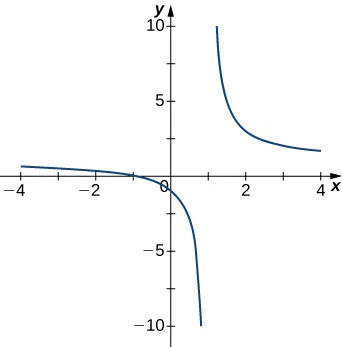252.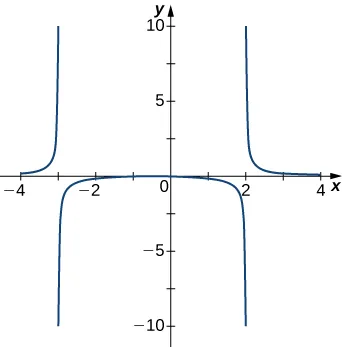253.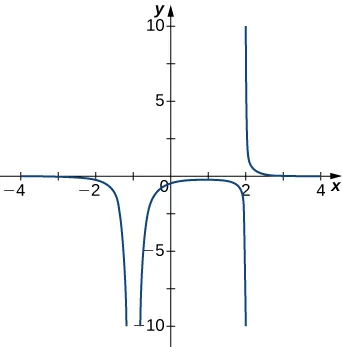254.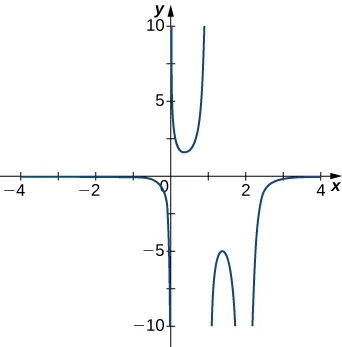255.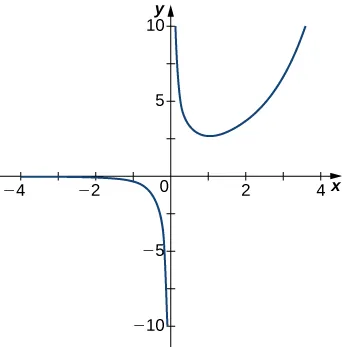For the following functions $f(x),f(x),$ determine whether there is an asymptote at $x=a.x=a.$ Justify your answer without graphing on a calculator.

256.

$f ( x ) = x + 1 x 2 + 5 x + 4 , a = −1 f ( x ) = x + 1 x 2 + 5 x + 4 , a = −1$

257.

$f ( x ) = x x − 2 , a = 2 f ( x ) = x x − 2 , a = 2$

258.

$f ( x ) = ( x + 2 ) 3 / 2 , a = −2 f ( x ) = ( x + 2 ) 3 / 2 , a = −2$

259.

$f ( x ) = ( x − 1 ) −1 / 3 , a = 1 f ( x ) = ( x − 1 ) −1 / 3 , a = 1$

260.

$f ( x ) = 1 + x −2 / 5 , a = 1 f ( x ) = 1 + x −2 / 5 , a = 1$

For the following exercises, evaluate the limit.

261.

$lim x → ∞ 1 3 x + 6 lim x → ∞ 1 3 x + 6$

262.

$lim x → ∞ 2 x − 5 4 x lim x → ∞ 2 x − 5 4 x$

263.

$lim x → ∞ x 2 − 2 x + 5 x + 2 lim x → ∞ x 2 − 2 x + 5 x + 2$

264.

$lim x → − ∞ 3 x 3 − 2 x x 2 + 2 x + 8 lim x → − ∞ 3 x 3 − 2 x x 2 + 2 x + 8$

265.

$lim x → − ∞ x 4 − 4 x 3 + 1 2 − 2 x 2 − 7 x 4 lim x → − ∞ x 4 − 4 x 3 + 1 2 − 2 x 2 − 7 x 4$

266.

$lim x → ∞ 3 x x 2 + 1 lim x → ∞ 3 x x 2 + 1$

267.

$lim x → − ∞ 4 x 2 − 1 x + 2 lim x → − ∞ 4 x 2 − 1 x + 2$

268.

$lim x → ∞ 4 x x 2 − 1 lim x → ∞ 4 x x 2 − 1$

269.

$lim x → − ∞ 4 x x 2 − 1 lim x → − ∞ 4 x x 2 − 1$

270.

$lim x → ∞ 2 x x − x + 1 lim x → ∞ 2 x x − x + 1$

For the following exercises, find the horizontal and vertical asymptotes.

271.

$f ( x ) = x − 9 x f ( x ) = x − 9 x$

272.

$f ( x ) = 1 1 − x 2 f ( x ) = 1 1 − x 2$

273.

$f ( x ) = x 3 4 − x 2 f ( x ) = x 3 4 − x 2$

274.

$f ( x ) = x 2 + 3 x 2 + 1 f ( x ) = x 2 + 3 x 2 + 1$

275.

$f ( x ) = sin ( x ) sin ( 2 x ) f ( x ) = sin ( x ) sin ( 2 x )$

276.

$f ( x ) = cos x + cos ( 3 x ) + cos ( 5 x ) f ( x ) = cos x + cos ( 3 x ) + cos ( 5 x )$

277.

$f ( x ) = x sin ( x ) x 2 − 1 f ( x ) = x sin ( x ) x 2 − 1$

278.

$f ( x ) = x sin ( x ) f ( x ) = x sin ( x )$

279.

$f ( x ) = 1 x 3 + x 2 f ( x ) = 1 x 3 + x 2$

280.

$f ( x ) = 1 x − 1 − 2 x f ( x ) = 1 x − 1 − 2 x$

281.

$f ( x ) = x 3 + 1 x 3 − 1 f ( x ) = x 3 + 1 x 3 − 1$

282.

$f ( x ) = sin x + cos x sin x − cos x f ( x ) = sin x + cos x sin x − cos x$

283.

$f ( x ) = x − sin x f ( x ) = x − sin x$

284.

$f ( x ) = 1 x − x f ( x ) = 1 x − x$

For the following exercises, construct a function $f(x)f(x)$ that has the given asymptotes.

285.

$x=1x=1$ and $y=2y=2$

286.

$x=1x=1$ and $y=0y=0$

287.

$y=4,y=4,$ $x=−1x=−1$

288.

$x = 0 x = 0$

For the following exercises, graph the function on a graphing calculator on the window $x=[−5,5]x=[−5,5]$ and estimate the horizontal asymptote or limit. Then, calculate the actual horizontal asymptote or limit.

289.

[T] $f(x)=1x+10f(x)=1x+10$

290.

[T] $f(x)=x+1x2+7x+6f(x)=x+1x2+7x+6$

291.

[T] $limx→−∞x2+10x+25limx→−∞x2+10x+25$

292.

[T] $limx→−∞x+2x2+7x+6limx→−∞x+2x2+7x+6$

293.

[T] $limx→∞3x+2x+5limx→∞3x+2x+5$

For the following exercises, draw a graph of the functions without using a calculator. Be sure to notice all important features of the graph: local maxima and minima, inflection points, and asymptotic behavior.

294.

$y = 3 x 2 + 2 x + 4 y = 3 x 2 + 2 x + 4$

295.

$y = x 3 − 3 x 2 + 4 y = x 3 − 3 x 2 + 4$

296.

$y = 2 x + 1 x 2 + 6 x + 5 y = 2 x + 1 x 2 + 6 x + 5$

297.

$y = x 3 + 4 x 2 + 3 x 3 x + 9 y = x 3 + 4 x 2 + 3 x 3 x + 9$

298.

$y = x 2 + x − 2 x 2 − 3 x − 4 y = x 2 + x − 2 x 2 − 3 x − 4$

299.

$y = x 2 − 5 x + 4 y = x 2 − 5 x + 4$

300.

$y = 2 x 16 − x 2 y = 2 x 16 − x 2$

301.

$y=cosxx,y=cosxx,$ on $x=[−2π,2π]x=[−2π,2π]$

302.

$y = e x − x 3 y = e x − x 3$

303.

$y = x tan x , x = [ − π , π ] y = x tan x , x = [ − π , π ]$

304.

$y = x ln ( x ) , x > 0 y = x ln ( x ) , x > 0$

305.

$y = x 2 sin ( x ) , x = [ −2 π , 2 π ] y = x 2 sin ( x ) , x = [ −2 π , 2 π ]$

306.

For $f(x)=P(x)Q(x)f(x)=P(x)Q(x)$ to have an asymptote at $y=2y=2$ then the polynomials $P(x)P(x)$ and $Q(x)Q(x)$ must have what relation?

307.

For $f(x)=P(x)Q(x)f(x)=P(x)Q(x)$ to have an asymptote at $x=0,x=0,$ then the polynomials $P(x)P(x)$ and $Q(x).Q(x).$ must have what relation?

308.

If $f′(x)f′(x)$ has asymptotes at $y=3y=3$ and $x=1,x=1,$ then $f(x)f(x)$ has what asymptotes?

309.

Both $f(x)=1(x−1)f(x)=1(x−1)$ and $g(x)=1(x−1)2g(x)=1(x−1)2$ have asymptotes at $x=1x=1$ and $y=0.y=0.$ What is the most obvious difference between these two functions?

310.

True or false: Every ratio of polynomials has vertical asymptotes.

Order a print copy

As an Amazon Associate we earn from qualifying purchases.